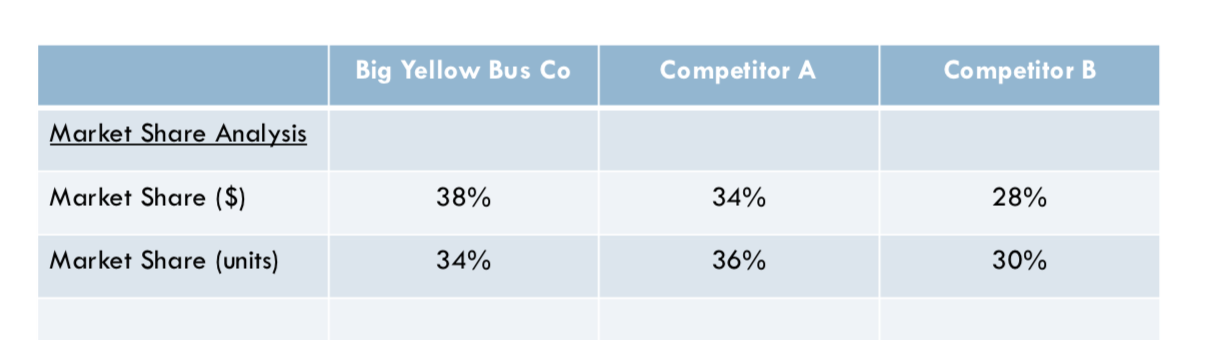1

# How to quickly calculate and analyze market share tables?

Dear all,

I stumbled upon this table in a case and I did figure out that prices for company BYB must be higher, but I think I took too long in calculating by how much the prices differ. Before I already realized that 40K buses are sold in the US and the market size in USD can be 6 billion USD.

However, calculating now for each the market in usd and units and then getting the price for each company does not only seem to take too much time, I also assume that interviewers expect a short cut here. I know that the short cut would be dividing the market share in usd percentage by the market share in units percentage for each company and then figuring out whats the percentage difference in price - BUT why is this so? Mathematically I can derive it, however, I still tend to take too much time.

Very happy to get some feedback on how to calculate such a table quickly using short cuts (and fast math) and also I would like to know (when dealing with market sizes tables) when I have to calculate the absolute numbers in order to compare it and when can I just compare percentages.

Thanks a lot!Dear all,

I stumbled upon this table in a case and I did figure out that prices for company BYB must be higher, but I think I took too long in calculating by how much the prices differ. Before I already realized that 40K buses are sold in the US and the market size in USD can be 6 billion USD.

However, calculating now for each the market in usd and units and then getting the price for each company does not only seem to take too much time, I also assume that interviewers expect a short cut here. I know that the short cut would be dividing the market share in usd percentage by the market share in units percentage for each company and then figuring out whats the percentage difference in price - BUT why is this so? Mathematically I can derive it, however, I still tend to take too much time.

Very happy to get some feedback on how to calculate such a table quickly using short cuts (and fast math) and also I would like to know (when dealing with market sizes tables) when I have to calculate the absolute numbers in order to compare it and when can I just compare percentages.

Thanks a lot!• Date ascending
• Date descending

Not sure whether I get the question...

So BYB has 38% \$-marketshare and 34%-unit share, then their \$-share is overindexed by (38/34 = 1.12 (approx.)). So if every bus costs 150k (6 bln / 40 k), then their busses cost 1.12 * 150k = 168 k.

To check whether this is correct, you can do the reverse math: 40 k buses * 34% = 13.6 k buses *168 k per bus = 2.2848 bln / 38% = 6.01 bln (a bit of a rounding error there, but you get the point)

Was that the question?

Not sure whether I get the question...

So BYB has 38% \$-marketshare and 34%-unit share, then their \$-share is overindexed by (38/34 = 1.12 (approx.)). So if every bus costs 150k (6 bln / 40 k), then their busses cost 1.12 * 150k = 168 k.

To check whether this is correct, you can do the reverse math: 40 k buses * 34% = 13.6 k buses *168 k per bus = 2.2848 bln / 38% = 6.01 bln (a bit of a rounding error there, but you get the point)

Was that the question?

## Related BootCamp article(s)

### Fast Math: How to Master Mental Math

Apply these tips and tricks for outstanding mental math shortcuts to impress your interviewer in your next case interview.

## Related case(s)

### Coronavirus Times - COVID-19 Brainteaser

Solved 700+ times